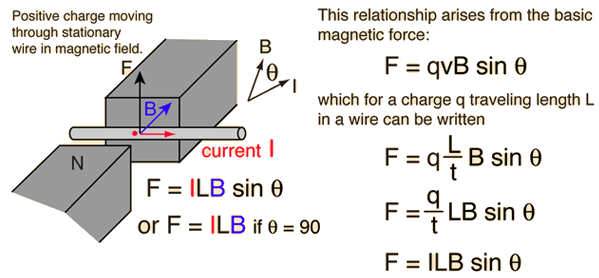Home » Ask Physics » Force on a current carrying conductor in a magnetic field

# Force on a current carrying conductor in a magnetic field

Why the force acting on current carrying conductor when placed in a magnetic field increases with the increase in the current.?

I mean what happens inside it at that time? Please explain it in detail, it’s really confusing me, Please answer it as sson as possible.

Let’s consider the terms involved here first.• Electric Current is the rate of flow of charges. An increase in current through a conductor means more number of electrons are crossing any cross section of the wire per second.
• A moving charge experience a force in magnetic field because a moving charge creates a magnetic field around it. The two interacting magnetic fields causes the force.

So, let’s summarize, when there is a current flowing through a conductor placed in a magnetic field, it experiences a force due to the mutual interaction between the magnetic fields – the already existing field and the field produced by the motion of current carriers (free electrons) inside the conductor.

When there is an increase in current, the force of interaction also increase as the numbers of free electrons flowing through any cross section also increases.

## 1 Comment

1. […] Force on a current carrying conductor in a magnetic field(askphysics.com) Related content: Force on a current carrying conductor in a magnetic field […]

This site uses Akismet to reduce spam. Learn how your comment data is processed.

### Hits so far @ AskPhysics

• 2,274,296 hits